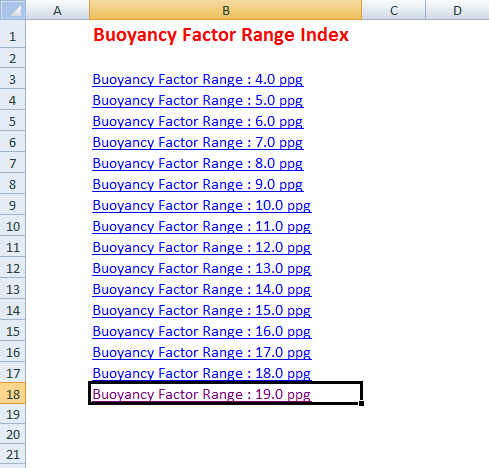Buoyancy factor is the factor that is used to compensate loss of weight due to immersion in drilling fluid and you can find the calculation from here https://www.drillingformulas.com/buoyancy-factor-calculation/.We have created a simple table to help people determine the buoyancy factor quickly. Let’s take a look at the table. In Figure 1, it shows the main page and you can select the mud weight range from 4.0 ppg to 19.0 ppg.Figure 1– Main Page BF Table

For instant, we choose 8.0 ppg and the table will show buoyancy from 8.0 – 8.9 ppg (Figure 2)Figure 2 – Buoyancy Factor for 8 ppg Range